Home » Class 10 » Important Questions for Class 10 Science Chapter 1 – Chemical Reactions and Equations

# Important Questions for Class 10 Science Chapter 1 – Chemical Reactions and Equations

This page contains Important Questions for Class 10 Science Chapter 1 - Chemical Reactions and Equations. In this page, you will find a collection of previous years board questions for this chapter along with their solutions. I am also giving the Important links below which can help you in further study of this chapter

Chemical reactions and equations class 10 Notes
Chemical Reactions and Equations NCERT Solutions
chemical reactions and equations class 10 questions answers
Chemical reactions and equations class 10 worksheet
Chemical reactions and equations class 10 test
Chemical reactions and equations class 10 mcq
NCERT Solutions for Class 10 Science


## Very Short answer type questions (1 marks each)

These types of questions usually contain 1 marks questions. You need to answer them in one word or one sentence.
Question 1. Which one is a chemical change-rusting of iron or melting of iron? [Board Term-I Set-11, 2011]
Question 2 Name and state the law which is kept in mind while we balance a chemical equation. [Board Term-I Set-21, 2011]
Answer. Law of conservation of mass. Mass can neither be created nor be destroyed during a chemical reaction.
Question 3. State one basic difference between a physical change and a chemical change. [Board Term-I Set-21, 2011]
Answer. In a physical change, no new substance is formed. In a chemical change, a new substance is formed.
Question 4. What happens when quicklime is added to water? [Board Term-I Set-Al, 2011, 2010]
Answer. Quicklime reacts with water vigorously to produce slaked lime and a large amount of heat.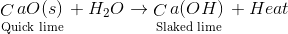Question 5. What happens when ZnCO3 is heated in the absence of air? Give the relevant equation. [Board Term-I Set-14, 2011]
Answer. $ZnO(s)$ and $CO_2(g)$ are formed. Chemical Equation: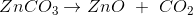Question 6. Is burning of a candle, a physical change or a chemical change ? [Board Term-I Set-33, 2011]
Answer. Both, chemical change and physical change.
Question 7. Write the chemical equation for reactions that take place when lead nitrate and potassium iodide solutions are mixed. [Board Term-I 2011]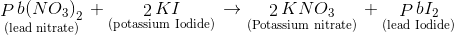Question 8. Write a balanced chemical equation :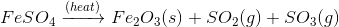[Board Term-I 2011]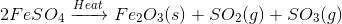Question 9. Which one is the chemical change – Fermentation of fruit juice or diluting fruit juice? [CBSE 2010]
Question 10. What is meant by the skeletal equation? [CBSE 2010]
Answer Unbalanced equation is called skeletal equation.
Question 11. Write the balanced chemical equation to represent the following reaction:
Carbon monoxide reacts with hydrogen gas at 340 atm. to form methyl alcohol. [CBSE 2010]
Answer.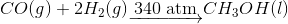Question 12. Write the type of reactions in the following:
(i) The reaction between an acid and a base
(ii) Rusting of iron
[CBSE, 2007]
(ii) Oxidation reaction

These questions are two Marks Questions and try to answer these questions in no more than 30 words.
Question 1 List four observations that help us to determine whether a chemical reaction has taken place. [Board Term-I, 2012]
Answer 1 (i) Evolution of gas
(ii) Change in temperature
(iii) Change in state
(iv) Change in color
Question 2. What is observed when carbon dioxide gas is passed through lime water.
(i) For a short duration
(ii) For long duration?
Also, write the chemical equations for the reaction involved. [Board Term I, 2016]
Answer 2. (i) For short duration :
Limewater turns milky due to the formation of $CaCO_3$, Which is insoluble in water.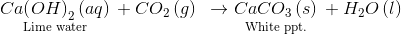(ii) For Long duration :
A clear solution is obtained due to the formation of calcium bicarbonate. $Ca (HCO_3)_2$ which is soluble in water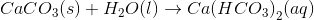Question 3 Write the balanced chemical equation for the following reaction and write the name of the reaction
Barium Chloride + Aluminium Sulphate $\rightarrow$ Barium Sulphate + Aluminium Chloride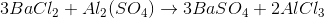It is a double displacement reaction.
Question 4 Why do we store silver chloride in dark colored bottles? Explain in brief. [CBSE 2010]
Answer 4. Silver chloride on exposure to sunlight may decompose as per the following reaction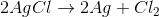Therefore it is stored in dark colored bottles.
Question 5. Define a combination reaction. Give one example of a combination reaction which is also exothermic.
Answer 5. A combination reaction is said to have occurred when two or more than two substances combine to form a single substance.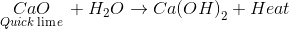Question 6. What happens when an iron nail is put inside the copper sulfate solution? Write a reaction with observation.
Answer 6. Iron nail turns brown, blue color of $CuSO_4$ changes to colorless.

$Fe+CuSO_4 \to FeS{{O}_{4}}+Cu$

Question 7 Name the raw materials that are required for the manufacture of washing soda by Solvay process. Describe the chemical reactions involved in the process. 
Answer 7. The raw materials needed for the manufacture of washing soda are $NaCl$ (sodium chloride), water, ammonia gas, and limestone to give $CO_2$ gas. Chemical reactions involved: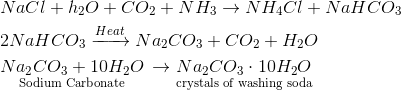Question 7 (i) State the law which is followed in balancing a chemical equation.
(ii) Balance the following chemical equation :

$Fe + H_2O \rightarrow Fe_30_4 + H_2$

[Board Term-I, 2012]
Answer 7 (i) Mass can neither be created nor destroyed in a chemical reaction-Law of conservation of mass.
(ii)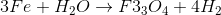Question 8 A white solid when dropped in water produces a hissing sound. What the solid may be? Give the chemical reaction for the above. Name the product formed.
Answer 8 White solid is quicklime $CaO$
Chemical reaction involved with the name of products is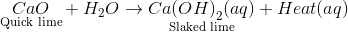Question 9 When Hydrogen gas is passed over heated copper (II) oxide, copper and steam are formed. Write the balanced chemical equation with physical states for this reaction. State what kind of chemical reaction is this?
[Board Term-I, 2015]
Answer 9.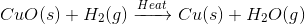This is a Redox reaction.

Questions worth practicing

(1) Reaction of carbon monoxide with heated copper oxide is a reduction. Explain.(2) What do you observe when concentrated sulphuric acid is added to sugar?

(3) What do you observe when zinc oxide is heated and then cooled?

(4) What do you observe when trilead tetraoxide is heated?

(5) What happens when ammonium carbonate is kept open?

(6) Why is the reaction between steam and red-hot iron said to be a reversible reaction?

(7) Give an example of a redox reaction involving a metallic oxide and a neutral gas as the only reactants.

(8) Classify the following reactions as exothermic and endothermic.

1. C+ O2—–> CO2
2. N+3H2—-> 2NH3
3. C + 2S —– > CS2
4. N2 + O2 —–> 2NO
5. CaCO3—- > CaO +CO2

(9) State the effect of:

a) An endothermic reaction

b) An exothermic reaction on the surrounding

(10) Predict the products for the following reactions and balance.

1. HCI + Zn—
2. NaBr + Cl2
3. H2SO4 + Fe—-
4. Na + H2O—-
5. Cu + AgNO3—-

(11) Complete the following double displacement reactions

1. KHO + H2SO4
2. NaOH + HCI—
3. Al(SO4)3 + NaOH—
4. BaCI+ Na2SO4
5. PbSO+ Na2CO3

(12) Classify the following reactions as thermal decomposition and thermal dissociation.

1. N2 + 3H2—- 2NH3
2. CaCO3— CaO +CO2
3. 2HgO—- 2Hg + O2
4. NH4 CI— NH3 + HCI

(13) Define a reversible reaction.

(14) Predict the type of each reaction.

1. 2Ag +S—-Ag2S
2. NH4NO2—-N2 + 2H2O
3. 2NAI + CI2—- 2NaCI + I2
4. CuCI+ 2NaOH—- Cu (OH)2 + 2NaCI

(15) How can nitrogen stop food items containing high fat and oil from turning rancid?

(16) Explains what happens when iron metal is left exposed in moist weather.

(17) (i) Why is aluminum used to reduce metal oxides like Fe2O3? (ii) What is “Thermite Welding”?

(18) How is iron ore reduced in the blast furnace?

(19) Why is Al obtained only by electrolytic reduction of Alumina (pure)?

(20) Name the two ores of zinc commonly used.

(21) Name two common alloys of zinc with their property and uses.

### 6 thoughts on “Important Questions for Class 10 Science Chapter 1 – Chemical Reactions and Equations”

1. Fe2o3+h2——-> 2fe +H20 + O2

2. CuO+H2 = Cu+H2O

1. Answer no.7 isnt balanced ????

This site uses Akismet to reduce spam. Learn how your comment data is processed.